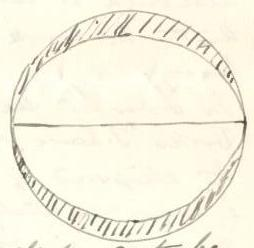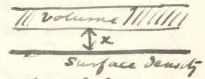# Transcription

## 3-15-17. George Howard Darwin to H. Poincaré

July 31 01

Newnham Grange–Cambridge

Dear Monsieur Poincaré,

I have now devised a method which I think overcomes all difficulties in carrying out my proposed work, although it certainly shows that the work will be very heavy.

In order to see how the process works I have applied it to the ellipsoid of revolution.11Darwin seeks to determine the energy lost in passing from a sphere to an ellipsoid of revolution, up to $e^{3}$, where $2e$ is the square of the eccentricity. He follows a similar procedure to get from the critical Jacobian ellipsoid to the pear-shaped figure. I unfortunately made a mistake in my work and have spent nearly fortnight in discovering where I was wrong. It was in an elementary point with which I need not trouble you, but I passed it by over and over again in going over my work.

If $2e$ is the square of the eccentricity of an ellipsoid of revolution of density $\rho$, mass $M$, and semi-major axis $a$, it is known that the “lost energy” is

 $\frac{3}{5}\frac{M^{2}}{a}\frac{\sin^{-1}\sqrt{2e}}{\sqrt{2e}}=\frac{3}{5}% \frac{M^{2}}{a}\left(1+\frac{1}{3}e+\frac{3}{10}e^{2}+\frac{5}{14}e^{3}\right)$

I want to get the same result by harmonic analysis.22In what follows, Darwin sums energies of the sphere and the layer to find the same expression for lost energy (up to $e^{3}$), an approach employed in Darwin (1887, 423).

I consider the ellipsoid to be built up by a sphere of radius $a$ and density $\rho$, and a (shaded) layer of density $-\rho$.

If

 $\displaystyle\sigma_{2}$ $\displaystyle=\frac{1}{3}-\mu^{2},$ $\displaystyle\sigma_{4}$ $\displaystyle=\mu^{4}-\frac{6}{7}\mu^{2}+\frac{3}{5\cdot 7}$ $\displaystyle\sigma_{6}$ $\displaystyle=\mu^{6}-\frac{3\cdot 5}{11}\mu^{4}+\frac{5}{11}\mu^{2}-\frac{5}{% 3\cdot 7\cdot 11}$

where $\mu$ is cosine of colatitude.The equation to the ellipsoid is33Darwin’s equation expresses the deformation of the figure with respect to the original sphere. Observing that $r=\sqrt{x^{2}+y^{2}+z^{2}}$ for a surface point $(x,y,z)$, we have $\left(\frac{r}{a}\right)^{2}=\frac{x^{2}}{a^{2}}+\frac{y^{2}}{a^{2}}+\frac{z^{% 2}}{a^{2}};$ thus, $\frac{r}{a}$ is precisely zero for surface points. Darwin builds here on an approach taken by Legendre and Laplace, who considered the layers of the figure as spheroids deviating from the sphere by small first-order quantities (Oppenheimer 1922). The equation of such a spheroid is $r=a(1+ef)$ where $r$ is the Euclidean distance from the origin to a surface point, $a$ is the radius of a sphere with center at the origin, $e$ is a small quantity with negligible square, and $f$ is a function of the polar coordinates $\theta$ and $\psi$ developed in a series of spherical functions $Y_{0}+Y_{1}+Y_{2}+\ldots$. Supposing $a$ to be the radius of the sphere with volume equal to the volume of the spheroid, one obtains $Y_{0}=0$, and by supposing the center of inertia to lie at the origin of the coordinate system, it is shown that $Y_{1}=0$. Laplace gave an expression for the equilibrium condition in terms of these functions, complicated by the consideration of supplementary forces. For the Earth, however, forces other than centrifugal may be neglected, and since the force expression involves only a spherical function of the second order, $P_{2}$, only $Y_{2}$ must be retained, and in $Y_{2}$, only the coefficient corresponding to $P_{2}=\cos^{2}\theta-\frac{1}{3}$ (the function labeled $\sigma_{2}$ by Darwin). Comparison with astronomical data indicated a need to introduce higher powers of $e$, motivating Darwin and others to consider more elaborate forms of the equation; see Wiechert (1897), Callandreau (1897), Darwin (1899).

 $\displaystyle\frac{r}{a}=1-\frac{1}{3}e-\frac{11}{2\cdot 3\cdot 5}e^{2}-\frac{% 103}{2\cdot 3\cdot 5\cdot 7}e^{3}\\ \displaystyle+\left(e+\frac{5}{7}e^{2}+\frac{9}{2\cdot 7}e^{3}\right)\sigma_{2% }+\frac{3}{2}\left(e^{2}+\frac{19}{11}e^{3}\right)\sigma_{4}-\frac{5}{2}e^{3}% \sigma_{6}\cdots{}$ (1)

I now form $\left(\frac{r}{a}\right)^{3}-\frac{1}{5}\left(\frac{r}{a}\right)^{5}$ and drop all terms which are of order higher than $e^{3}$ or which will vanish when integrated over the sphere. Taking this expression between the limits $a$ and $r$ as given in (A), I find

 $\left[\frac{r^{3}}{a^{3}}-\frac{1}{5}\frac{r^{5}}{a^{5}}\right]^{a}_{r}=\frac{% 2}{3}e+\frac{28}{3^{2}\cdot 5}e^{2}+\frac{661}{3^{2}\cdot 5\cdot 7}e^{3}-\left% (e^{2}+\frac{17}{7}e^{3}\right)(\sigma_{2})^{2}+e^{3}(\sigma_{2})^{3}.$

It is easy to show that

 $\begin{matrix}\iint d\mu d\phi=4\pi;&\iint(\sigma_{2})^{2}d\mu d\phi=\frac{16% \pi}{45};&\iint(\sigma_{2})^{3}d\mu d\phi=-\frac{64\pi}{3^{3}\cdot 5\cdot 7}.% \end{matrix}$

Hence I find

 $\iint\left[\frac{r^{3}}{a^{3}}-\frac{1}{5}\frac{r^{5}}{a^{5}}\right]^{a}_{r}d% \mu d\phi=\frac{8\pi}{3}\left(e+\frac{4}{5}e^{2}+\frac{7}{10}e^{3}\right).$

Now to make use of this.

The lost energy of the sphere, say $E_{1}$, is

 $\frac{3}{5}\frac{\left(\frac{4}{3}\pi\rho a^{3}\right)^{2}}{a}=\frac{16}{15}% \pi^{2}\rho^{2}a^{5}$

The lost energy of the sphere and layer, say $E_{2}$, is the potl of the sphere multiplied by density of layer and integrated through the layer. Hence it is

 $-\iiint\frac{2}{3}\pi\rho(3a^{2}-r^{2})\rho r^{2}drd\mu d\phi$

with the limits of $r$: $a$ to $r$ (as above).

But

 $\int_{r}^{a}\left(3\frac{r^{2}}{a^{3}}-\frac{r^{4}}{a^{5}}\right)dr=\left[% \frac{r^{3}}{a^{3}}-\frac{1}{5}\frac{r^{5}}{a^{5}}\right]^{a}_{r}$

Hence applying the proceeding result

 $E_{1}+E_{2}=\frac{16}{15}\pi^{2}\rho^{2}a^{5}\left(1-\frac{5}{3}e-\frac{4}{3}e% ^{2}-\frac{7}{6}e^{3}\right)$

Now since44For comparison, Thomson & Tait (1879, § 776, eq. 7) found $M=\frac{4}{3}\pi\rho a^{3}\frac{1}{1-2e}$.

 $M=\frac{4}{3}\pi\rho a^{3}\sqrt{1-2e}$

We have, on division by $1-2e$

 $E_{1}+E_{2}=\frac{3}{5}\frac{M^{2}}{a}\left(1+\frac{1}{3}e-\frac{2}{3}e^{2}-% \frac{5}{2}e^{3}\right)$

This result may be obtained more shortly, but I have purposely done it as if I was compelled to integrate.

It remains to find the lost energy of the layer. This I consider in three steps.

• 1.

Concentrate the layer on the sphere and find its lost energy $E_{3}$

• 2.

Distort the layer of surface density by carrying it to a position halfway between the sphere and ellipsoid, and let $E_{4}$ be the energy lost

• 3.

Expand the surface density until it fills the layer, and let $E_{5}$ (essentially negative) be the lost energy.

(1) The equation to the ellipsoid (retaining squares only) may be written55Variant after first equation: “$-e^{3}(4\mu^{2}-6\mu^{4})$”.

 $\displaystyle\frac{r}{a}$ $\displaystyle=1-e\mu^{2}-e^{2}\left(2\mu^{2}-\frac{3}{2}\mu^{4}\right).$ $\displaystyle\frac{r^{3}}{a^{3}}$ $\displaystyle=1-3\mu^{2}(e+2e^{2})+\frac{15}{2}e^{2}\mu^{4}$ $\displaystyle=1-e-\frac{1}{2}e^{2}+3\sigma_{2}\left(e-\frac{1}{7}e^{2}\right)+% \frac{15}{2}e^{2}\sigma_{4}$ $\displaystyle\frac{1}{3}\left[\frac{r^{3}}{a^{3}}\right]^{r}_{a}$ $\displaystyle=\frac{1}{3}e+\frac{1}{6}e^{2}-\sigma_{2}\left(e-\frac{1}{7}e^{2}% \right)-\frac{5}{2}e^{2}\sigma_{4}$

The mass which stands on the element $a^{2}d\mu d\phi$ is

 $-\int^{a}_{r}\rho\frac{r^{2}}{a^{2}}dr=-\rho a\left[\frac{1}{3}e+\frac{1}{6}e^% {2}-\sigma_{2}\left(e-\frac{1}{7}e^{2}\right)-\frac{5}{2}e^{2}\sigma_{4}\right]$

and this is the surface density of the concentration (1). Proceeding in the usual way to find the lost energy

 $\displaystyle E_{3}$ $\displaystyle=\frac{16}{15}\pi^{2}\rho^{2}a^{5}\left(\frac{29}{2\cdot 3\cdot 5% }e^{2}+\frac{167}{2\cdot 3\cdot 5\cdot 7}e^{3}\right)$ $\displaystyle=\frac{3}{5}\frac{M^{2}}{a}\left(\frac{29}{2\cdot 3\cdot 5}e^{2}+% \frac{191}{2\cdot 5\cdot 7}e^{3}\right)$ Then $\displaystyle E_{1}+E_{2}+E_{3}$ $\displaystyle=\frac{3}{5}\frac{M^{2}}{a}\left(1+\frac{1}{3}e+\frac{3}{10}e^{2}% +\frac{8}{5\cdot 7}e^{3}\right)$

This is already right up to $e^{2}$.

(2) To the first order of small quantities, the surface density of the concentration is

 $\rho ae\left(\sigma_{2}-\frac{1}{3}\right)$

The external and internal potentials are

 $\displaystyle V_{0}$ $\displaystyle=4\pi\rho a^{2}e\left(\frac{1}{5}\frac{a^{3}}{r^{3}}\sigma_{2}-% \frac{1}{3}\frac{a}{r}\right)$ $\displaystyle V_{i}$ $\displaystyle=4\pi\rho a^{2}e\left(\frac{1}{5}\frac{r^{2}}{a^{2}}\sigma_{2}\right)$

The mean value of $\frac{dV_{0}}{dr}$ and $\frac{dV_{i}}{dr}$ $(r=a)$ is then

 $2\pi\rho ae\left(-\frac{1}{5}\sigma_{2}+\frac{1}{3}\right)$

This represents the attraction on an element of the surface which bears density $\rho ae(\sigma_{2}-\frac{1}{3})$, and is to be carried a distance $\frac{1}{2}ae(\sigma_{2}-\frac{1}{3})$.

Hence

 $\displaystyle E_{4}$ $\displaystyle=\iint 2\pi\rho ae\left(\frac{1}{3}-\frac{1}{5}\sigma_{2}\right)% \cdot\frac{1}{2}\rho\cdot a^{2}e^{2}\left(\sigma_{2}-\frac{1}{3}\right)^{2}a^{% 2}d\mu d\varphi$ $\displaystyle=\pi\rho^{2}a^{5}e^{3}\iint\mu^{4}\left(\frac{1}{3}-\frac{1}{3% \cdot 5}+\frac{1}{5}\mu^{2}\right)d\mu d\varphi$ $\displaystyle=\frac{4\pi^{2}\rho^{2}a^{5}e^{3}}{5}\left(\frac{1}{7}+\frac{4}{3% \cdot 5}\right)$ $\displaystyle=\frac{3}{5}\frac{M^{2}}{a}\left(\frac{43e^{3}}{4\cdot 5\cdot 7}\right)$

And

 $E_{1}+E_{2}+E_{3}+E_{4}=\frac{3}{5}\frac{M^{2}}{a}\left(1+\frac{1}{3}e+\frac{3% }{10}e^{2}+\frac{15}{4\cdot 7}e^{3}\right)$

(3) Consider a plane surface coated with surface density $2h\rho$, and find the gain of energy in expanding it to be a slab of thickness $2h$ — half being carried one way and half the other.

Imagine the expansion of one half carried out until $h-x$ is filled with density $\rho$ & $h+x$ remains unexpanded. The force per unit area is66For the right-hand side, read $-4\pi\rho x$. $2\pi\rho(h-x)-2\pi\rho x-2\pi\rho h=-4\pi\rho hx.$The next element carried up has mass $\rho dx$ & is carried thro’ a distance $x$. Hence the loss of energy in the whole process is

 $2\int^{h}_{0}2\pi\rho^{2}x^{2}dx$

per unit area or $\frac{1}{3}\pi\rho^{2}(\text{thickness})^{3}$.

In the case we are now considering the thickness is $ae(\sigma_{2}-\frac{1}{3})$.

Thus

 $\displaystyle E_{5}$ $\displaystyle=\frac{1}{3}\pi\rho^{2}\iint a^{3}e^{3}\left(\sigma_{2}-\frac{1}{% 3}\right)^{3}a^{2}d\mu d\varphi$ $\displaystyle=-\frac{1}{3}\pi\rho^{2}a^{5}e^{3}\iint\mu^{6}d\mu d\varphi$ $\displaystyle=-\frac{4\pi^{2}\rho^{2}a^{5}e^{3}}{3\cdot 7}$ $\displaystyle=-\frac{3}{5}\frac{M^{2}}{a}\left(\frac{5}{4\cdot 7}e^{3}\right).$

 $E_{1}+E_{2}+E_{3}+E_{4}+E_{5}=\frac{3}{5}\frac{M^{2}}{a}\left(1+\frac{1}{3}e+% \frac{3}{10}e^{2}+\frac{5}{14}e^{3}\right).$

This is the correct result.

You will see that the whole of this can be done with Jacobi’s ellipsoid & the pear, but if this is somewhat laborious how much more will that be so.77Gharnati (1996, 67, n. 92) recovers Darwin’s result starting from MacLaurin’s exact formula.

Yours sincerely,

G. H. Darwin

ALS 8p. Collection particulière, Paris 75017.

## References

• O. Callandreau (1897) Sur la théorie de la figure des planètes; applications à Jupiter et à la Terre. Bulletin astronomique 14, pp. 214–217. External Links: Link
• G. H. Darwin (1887) On figures of equilibrium of rotating masses of fluid. Philosophical Transactions of the Royal Society of London 178, pp. 379–428.
• G. H. Darwin (1899) The theory of the figure of the Earth carried to the second order of small quantities. Monthly Notices of the Royal Astronomical Society 60, pp. 82–124. External Links: Link
• A. Gharnati (1996) La correspondance entre Henri Poincaré et George Howard Darwin : Origine et stabilité des figures piriformes). Ph.D. Thesis, Université Nancy 2, Nancy.
• S. Oppenheim (1922) Die Theorie der Gleichgewichtsfiguren der Himmelskörper. In Encyklopädie der mathematischen Wissenschaften mit Einschluss ihrer Anwendungen, Bd. 6, Astronomie, Teil 2B, S. Oppenheim and W. Dyck (Eds.), pp. 1–79. External Links: Link
• W. Thomson and P. G. Tait (1879) Treatise on Natural Philosophy. 2d edition, Cambridge University Press, Cambridge. External Links: Link
• E. Wiechert (1897) Ueber die Massenverteilung im Inneren der Erde. Nachrichten von der Königlichen Gesellschaft der Wissenschaften zu Göttingen, mathematisch-physikalische Klasse, pp. 221–243. External Links: Link

#### Titre (dcterms:title)

George Howard Darwin à Henri Poincaré, 31 juillet 1901

#### Incipit (ahpo:incipit)

I have now devised a method which I think ...

1901-07-31

#### Type (ahpo:documentType)

fr Lettre autographe signée

17

CD n° 114

#### Droits (ahpo:rightsHolder)

Archives Henri Poincaré

8

en

Gharnati Ph.D.